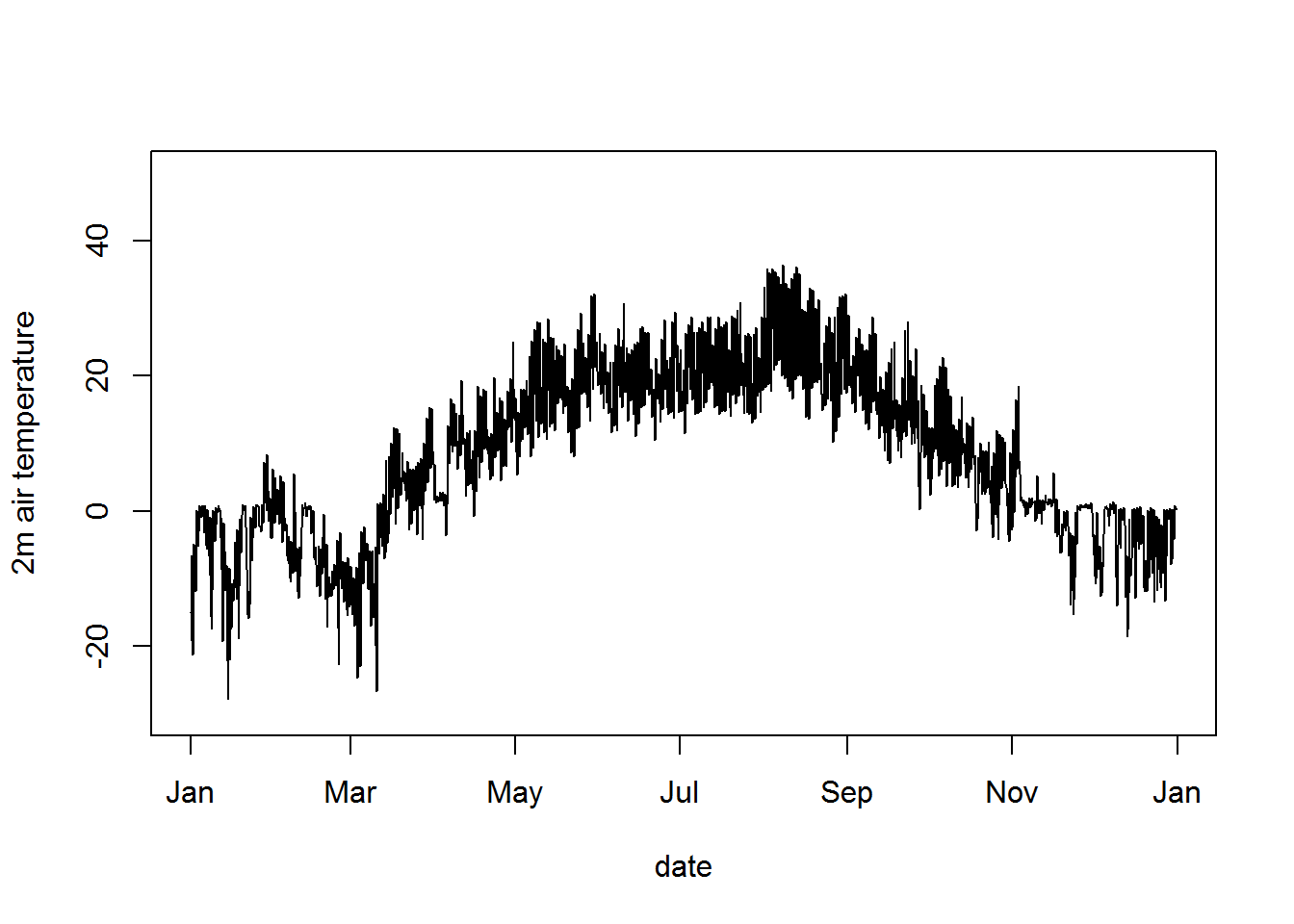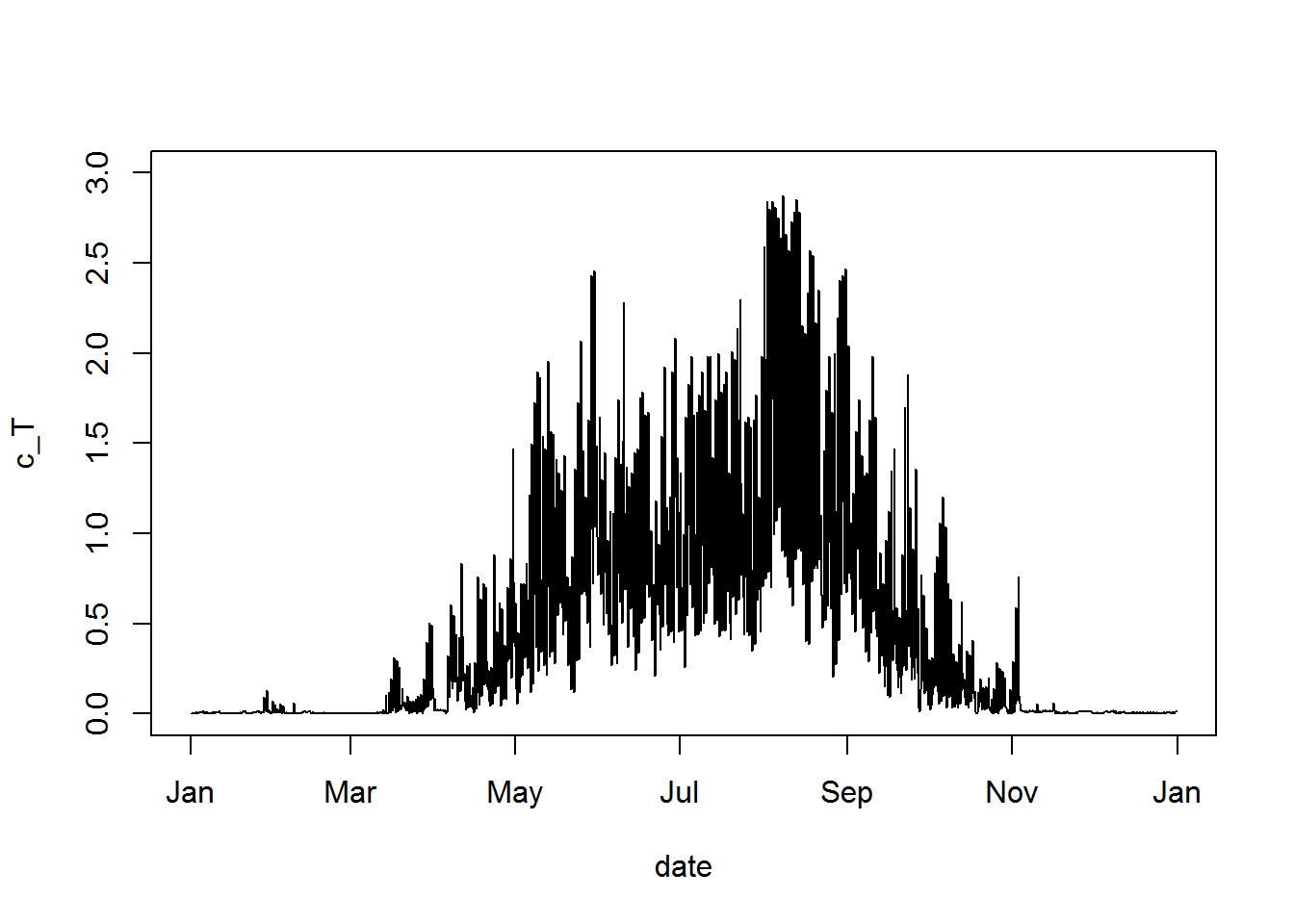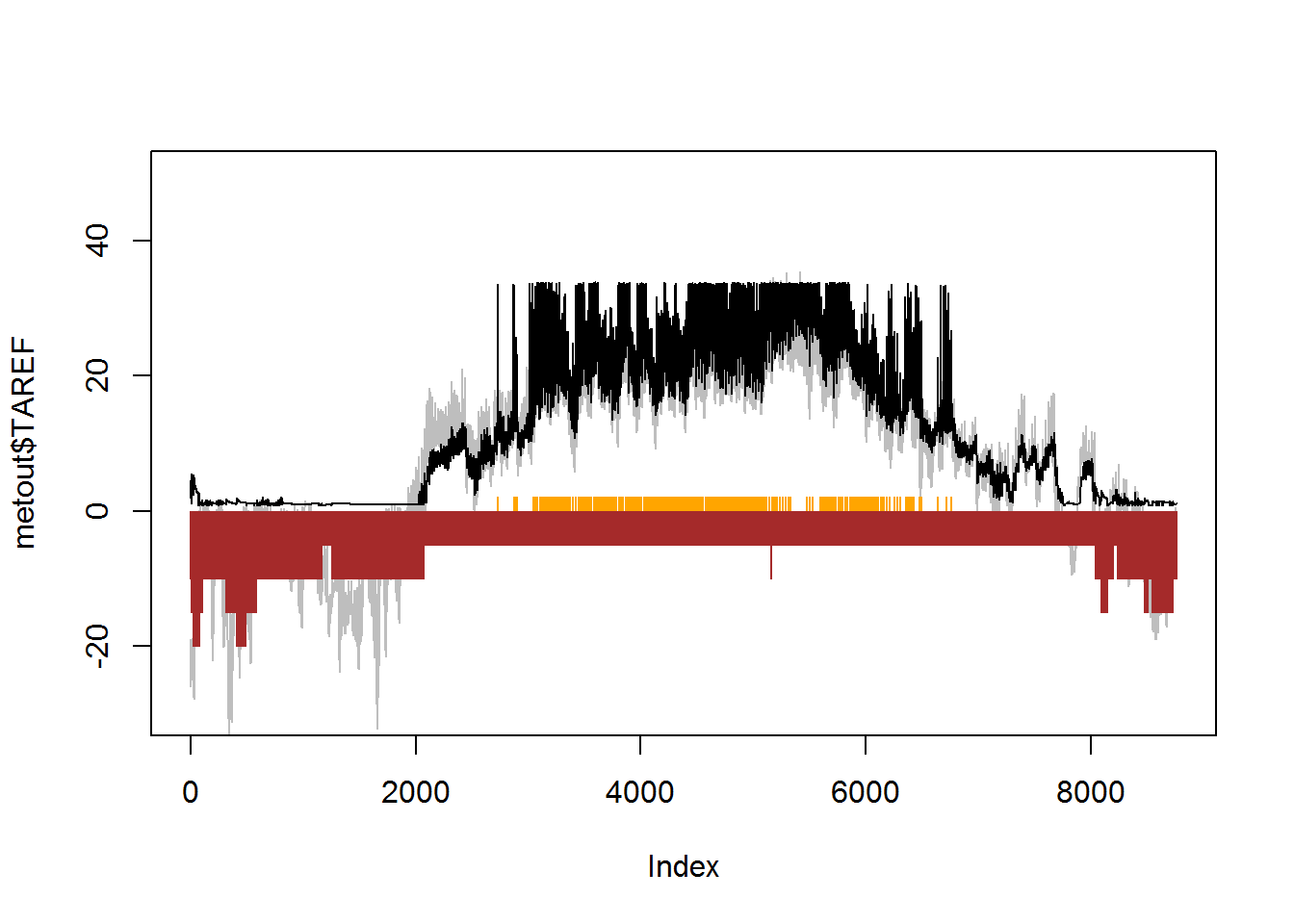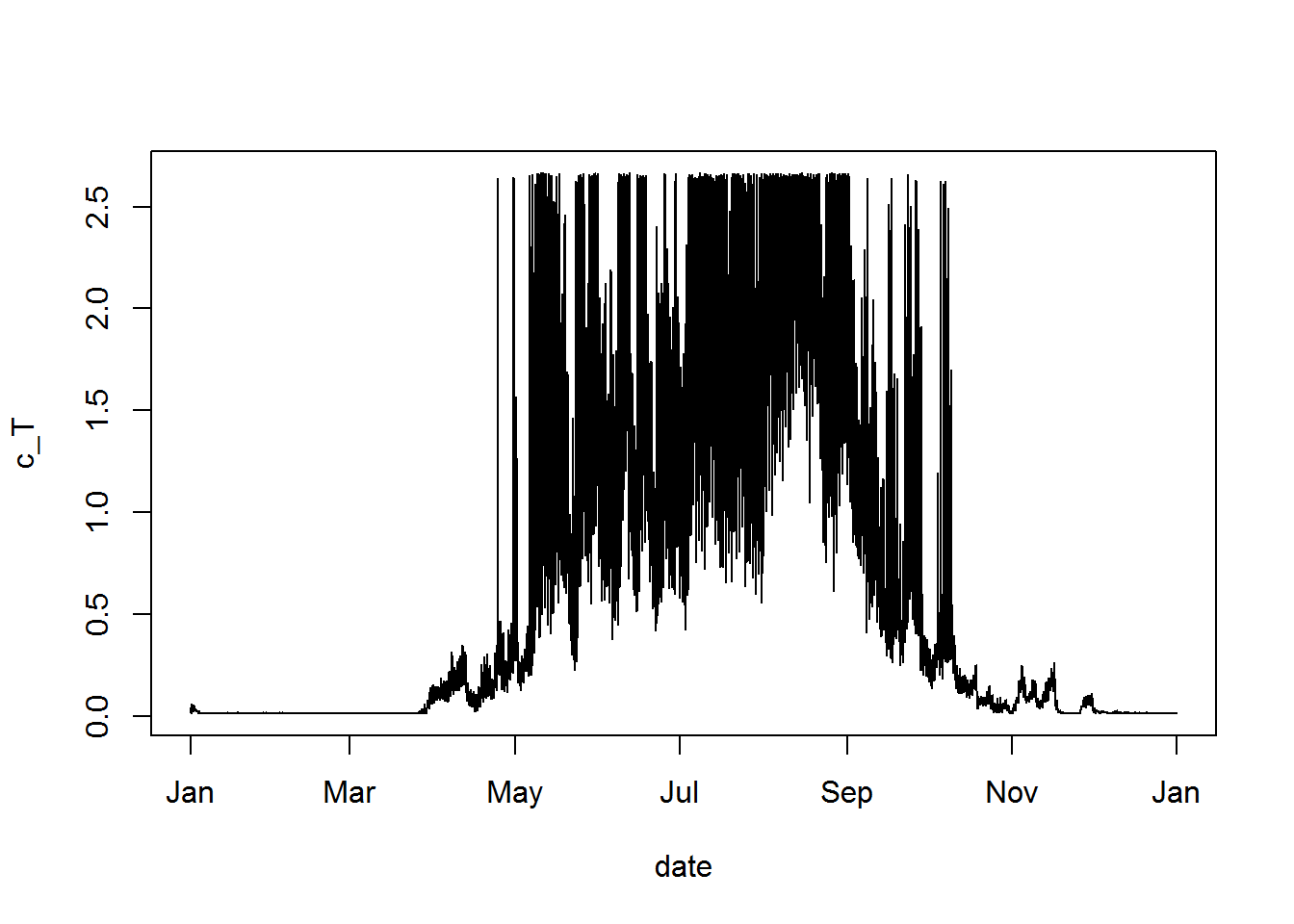### Overview

In DEB parameter estimation it is critical to get a good estimate of the body temperature at which growth, reproduction, development and aging rates are observed. A measure of mean ‘ambient’ temperature will often be inaccurate, leading to poor parameter estimates. For example, often the observations of these rates are made for organisms experiencing fluctuating body temperatures, as typically occurs in nature or if animals are cultured under cycling temperature regimes. Moreover, the temperatures experienced may be above ambient air temperature if the organism is in the boundary layer near the ground, exposed to sunlight or high levels of infrared radiation. It may also be below air temperature if it is cooling by evaporation (e.g. a frog) or to cold radiative environemnts (e.g. clear night skies).

This document shows how to estimate the body temperatures of animals in nature by two methods of increasing sophistication. First, it shows how to extract 6-hourly air temperature data for a location of interest (e.g. from where field growth rates have been observed) from a global database of historical daily weather data via the RNCP package (Kemp et al. 2012). Second it shows how to use the NicheMapR package to estimate microclimates (e.g. near-ground air temperatures, soil temperatures) and to simulate heat exchange for an organism of given physical characteristics (e.g. solar reflectance) and behavioural thermoregulatory responses.

In each case the resulting temperature vector is converted to a ‘constant temperature equivalent’, CTE, which can be used as the temperature estimate for zero-variate observations of e.g. age at birth, age at puberty, longevity and reproduction rate.

For the example we will use the sand lizard, Lacerta agilis, which is in the AmP collection and has field growth observations for a number of locations in Russia (Roitberg and Smirina 2006).

### Package Installation

First install and load the necessary packages (if you don’t have them already).

install.packages("rvest")
install.packages("RNCEP")
install.packages("ncdf4")
install.packages("devtools")
install.packages("stringr")
devtools::install_github("mrke/NicheMapR")  # you can also download a binary from https://github.com/mrke/NicheMapR/releases/

library(rvest)
## Loading required package: xml2
library(RNCEP)
## Loading required package: maps
library(ncdf4)
library(stringr)
library(NicheMapR)

### Extracting NCEP air temperature for a location and time

The RNCEP package interfaces with two gridded (~2.5 degree) global data set on various meteorological variables that go back to as far as 1957 and as recently as the past month. You can read more about these data sets in (Kemp et al. 2012).

Here we are quering the ‘air.2m’ variable, which is the estimate of air temperature at the typical weather station height. There are a number of other options you could choose.

We need to specify the location as longitude and latitude (decimal degrees), as well as the starting and ending year and the starting and ending months. Note that you ONLY get the months you ask for, so if you’re trying to get a continuous period that spans a year, you should do separate queries per year and combine them.

# define query inputs
lonlat <- c(46.8, 43.3)  # the Kostek site of Roitberg and Smirina (2006)
y.start <- 1985
y.finish <- 1985
m.start <- 1
m.finish <- 12
var <- "air.2m"  # you could also choose e.g. 'skt.sfc' (surface temperature) or 'tmp.0-10cm' (shallow soil)
lev <- "gaussian"

# run the query
tair <- NCEP.gather(variable = var, level = lev, months.minmax = c(m.start,
m.finish), years.minmax = c(y.start, y.finish), lat.southnorth = c(lonlat,
lonlat), lon.westeast = c(lonlat, lonlat), reanalysis2 = FALSE,
return.units = TRUE, status.bar = FALSE)
##  Units of variable 'air.2m' are degK
# extract the actual coordinates you got data from (given
# that the grid is coarse)
lonlat.returned <- c(as.numeric(dimnames(tair)[]), as.numeric(dimnames(tair)[]))

# dates
dates1 <- seq(ISOdate(y.start, m.start, 1, tz = "UTC") - 3600 *
12, ISOdate(y.finish + 1, 1, 1, tz = "UTC") - 3600 * 13,
by = "hours")
dates <- dates1[seq(1, length(dates1), 6)]  # cut down to 6-hourly

t.air <- cbind(as.data.frame(dates), tair[1, 1, ])
colnames(t.air) <- c("date", var)
plot(t.air - 273.15, type = "l", ylim = c(-30, 50), ylab = "2m air temperature")  # plot, converting to deg C# write to disk if you want write.csv(tair[1, 1, ],
# 'tair.csv')

### Compute constant temperature equivalent (CTE) based on air temperature

Now we want to compute a ‘constant temperature equivalent’, CTE, which is an estimate of the mean temperature accounting for the nonlinearity of the thermal response curve.

First, some parameters are defined for the 5-parameter Arrhenius response curve. These are a rough guess for Lacerta agilis.

T_A <- 8000
T_AL <- 20000
T_AH <- 20000
T_L <- 10 + 273.15
T_H <- 40 + 273.15
T_REF <- 20 + 273.15

Now we define the temperature vector of interest, T, as the 2 m air temperature NCEP data, and use the 5 parameter Arrhenius formula to convert it to the temperature correction factor, c_T.

T <- t.air$air.2m # temps from simulation to be used to estimate CTE Tjuly <- mean(T[which(format(dates, "%m") == "07")] - 273.15) # get mean July air temperature # convert Tb each hour to temperature correction factor c_T <- as.numeric(exp(T_A * (1/T_REF - 1/T))/(1 + exp(T_AL * (1/T - 1/T_L)) + exp(T_AH * (1/T_H - 1/T)))) plot(c_T ~ t.air$date, type = "l", xlab = "date", ylim = c(0,
3))Now we compute the mean c_T and define a function for use in iteratively guessing values of body temperature that result in this mean c_T value, i.e. we turn the mean temperature correction factor back into a temperature. The ‘uniroot’ function is used to do the guessing, with bracketing starting T_L - 10 and T_H + 10.

c_T.mean <- mean(c_T)  # get mean temperature correction factor

getCTE <- function(T_b) {
# function finding the difference between a temperature
# correction factor, c_T.mean, for a specified T_b compared
# to the mean calculated one (aim to make this zero)
x <- exp(T_A * (1/T_REF - 1/T_b))/(1 + exp(T_AL * (1/T_b -
1/T_L)) + exp(T_AH * (1/T_H - 1/T_b))) - c_T.mean
}
# search for a Tb (CTE) that gives the same temperature
# correction factor as the mean of the simulated temperature
# corrections
CTE <- uniroot(f = getCTE, c(T_L - 10, T_H + 10), check.conv = TRUE)$root - 273.15 # converting from K to deg C We now have the constant temperature equivalent. As you can see from the values below, the naïve temperature correction factor we’d get from the mean of the raw input air temperatures (8.1 °C) is around 0.1 but transformation of the 6-hourly temperatures into c_T gives a mean of 0.45. This corresponds to a CTE of 14.5 °C, i.e. over 6 °C higher than the mean air temperature. Note that we also get a mean July air temperature of ~21 °C which is a bit lower than the value of 24 °C reported in Roitberg and Smirina (2006). { cat(paste0("mean T_air = ", round(mean(T), 2) - 273.15), "\n") # report value to console cat(paste0("mean T_air, July = ", round(Tjuly, 2)), "\n") # report value to console cat(paste0("mean c_T = ", round(c_T.mean, 2)), "\n") # report value to console cat(paste0("naïve c_T = ", round(as.numeric(exp(T_A * (1/T_REF - 1/mean(T)))/(1 + exp(T_AL * (1/mean(T) - 1/T_L)) + exp(T_AH * (1/T_H - 1/mean(T))))), 2)), "\n") # report value to console cat(paste0("CTE = ", round(CTE, 2)), "\n") # report value to console } ## mean T_air = 8.09000000000003 ## mean T_air, July = 20.76 ## mean c_T = 0.45 ## naïve c_T = 0.12 ## CTE = 14.52 ### Using NicheMapR to estimate body temperature including thermoregulatory behaviour The use of fluctuating air temperature is clearly making a difference to the temperature we would associate with field observations for this lizard. The next section shows an example of how to do this using the NicheMapR package (Kearney and Porter, 2017). This package includes a microclimate model, which computes aboveground and belowground conditions relevant to solving heat and water budgets of organisms (radiation, wind, humidty, air temperature). It also includes an ectotherm model of heat and water exchange in the context of behavioural regulation under spatially implicit microclimatic variation (Kearney et al. 2012; Kearney et al. 2018). I.e. extremes of available shade, and the range of possible depths, are provided as well as body temperature thresholds for different behavioural responses, and the model computes an hourly temperature trajectory give the microclimate. Here we run the microclimate function ‘micro_ncep’ which forces the microclimate model via the RNCEP package used above, but also extracting information on humidity, wind speed, cloud cover, air pressure and solar radiation from the NCEP data. dstart <- "01/01/1985" dfinish <- "31/12/1985" micro <- micro_ncep(loc = lonlat, dstart = dstart, dfinish = dfinish) ## Loading required package: sp ## extracting weather data with RNCEP ##  Units of variable 'air.2m' are degK ##  Units of variable 'prate.sfc' are Kg/m^2/s ##  Units of variable 'shum.2m' are kg/kg ##  Units of variable 'pres.sfc' are Pascals ##  Units of variable 'tcdc.eatm' are % ##  Units of variable 'uwnd.10m' are m/s ##  Units of variable 'vwnd.10m' are m/s ##  Units of variable 'dswrf.sfc' are W/m^2 ## running microclimate model for 365 days from 01/01/1985 to 31/12/1985 at site long 46.8 lat 43.3 ## user system elapsed ## 14.01 0.07 14.12 Next we have to specify the parameters determining how the species behaviourally thermoregulates. Brief explanations are provided next to each one. Ww_g <- 30 # wet weight, grams CT_max <- 42 # critical thermal maximum CT_min <- 0.7 # critical thermal minimum T_F_max <- 40 # maximum Tb at which activity occurs T_F_min <- 25 # minimum Tb at which activity occurs T_pref <- 33.8 # preferred Tb (will try and regulate to this) T_B_min <- 20 # minimum Tb at which leaves retreat T_RB_min <- 15 # minimum Tb at which they will attempt to leave retreat shape <- 3 # shape (3 = lizard) shade_seek <- 1 # shade seeking? burrow <- 1 # can it move below ground? minshade <- 0 # minimum available shade? mindepth <- 3 # minimum depth (node, 1-10) allowed maxdepth <- 10 # maxmaximum depth (node, 1-10) allowed nocturn <- 0 # nocturnal activity? crepus <- 0 # crepuscular activity? diurn <- 1 # diurnal activity? Finally we run the ectotherm model and plot the results. In the figure you can see in grey the air temperature, as we extracted via RNCEP above, but also in black the predicted temperature incorporating the thermoregulatory response, which includes moving underground to escape extremes of heat and cold, and choosing shade to attempt to stay close to the preferred range. In brown is the depth selected (cm) and the orange indicates the hours foraging was possible. You can see the lizard is warmer in the winter due to being underground and under snow, and can also get warmer in the active season due to exposure to solar radiation. ecto <- ectotherm(nocturn = nocturn, diurn = diurn, shape = shape, T_F_max = T_F_max, T_F_min = T_F_min, T_pref = T_pref, T_B_min = T_B_min, T_RB_min = T_RB_min, CT_max = CT_max, CT_min = CT_min, crepus = crepus, burrow = burrow, shade_seek = shade_seek, mindepth = mindepth, maxdepth = maxdepth, minshade = minshade) ## running ectotherm model ... ## runtime 0.620000000000005 seconds environ <- as.data.frame(ecto$environ)
metout <- as.data.frame(micro$metout) # plot some results plot(metout$TAREF, ylim = c(-30, 50), type = "l", col = "grey")
points(environ$TC, type = "l") points(environ$ACT, type = "h", col = "orange")
points(environ$DEP, type = "h", col = "brown")Now we repeate the procedure above for getting the CTE, using estimated body temperature as our input vector. T <- environ$TC + 273.15  # temps from simulation to be used to estimate CTE
# convert Tb each hour to temperature correction factor
c_T <- as.numeric(exp(T_A * (1/T_REF - 1/T))/(1 + exp(T_AL *
(1/T - 1/T_L)) + exp(T_AH * (1/T_H - 1/T))))
plot(c_T ~ dates1, type = "l", xlab = "date")c_T.mean <- mean(c_T)  # get mean temperature correction factor
# search for a Tb (CTE) that gives the same temperature
# correction factor as the mean of the simulated temperature
# corrections
CTE <- uniroot(f = getCTE, c(T_L - 10, T_H + 10), check.conv = TRUE)\$root -
273.15  # converting from K to deg C
Tjuly <- mean(T[which(format(dates1, "%m") == "07")] - 273.15)
{
cat(paste0("mean T_b = ", round(mean(T), 2) - 273.15), "\n")  # report value to console
cat(paste0("mean T_b, July = ", round(Tjuly, 2)), "\n")  # report value to console
cat(paste0("mean c_T = ", round(c_T.mean, 2)), "\n")  # report value to console
cat(paste0("naïve c_T = ", round(as.numeric(exp(T_A * (1/T_REF -
1/mean(T)))/(1 + exp(T_AL * (1/mean(T) - 1/T_L)) + exp(T_AH *
(1/T_H - 1/mean(T))))), 2)), "\n")  # report value to console
cat(paste0("CTE = ", round(CTE, 2)), "\n")  # report value to console
Tjuly <- mean(T[which(format(dates1, "%m") == "07")] - 273.15)
}
## mean T_b = 12.5
## mean T_b, July = 26.71
## mean c_T = 0.66
## naïve c_T = 0.32
## CTE = 17.3

When we incorporate behaviour, we end up with a higher raw input temperature of 12.5 °C and a higher CTE of 17.3 °C. Roitberg and Smirina (2006) reported a mean July air temperature of 24 °C, which was the value used in the original Add My Pet entry for this species. It would be more appropriate, however, to use a value of 17.3 °C incorporating the nonlinear rate effects and considering the whole year because the growth curves are over multiple years. This would result in parameters permitting more rapid growth in cold climates.